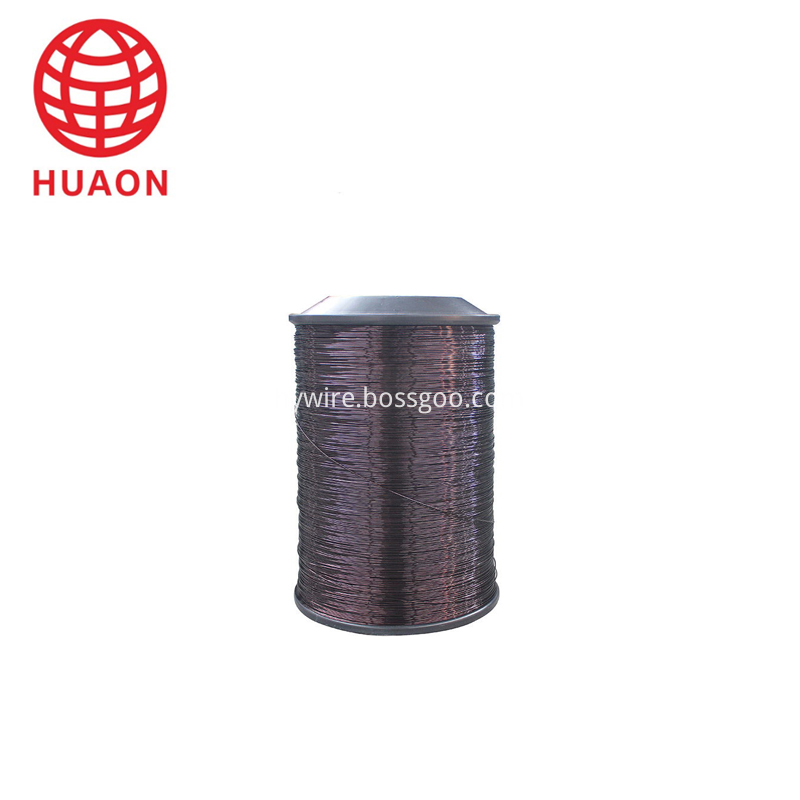# Sound pressure level calculation method for indoor sound reinforcement

In the design of indoor sound reinforcement field, the state has relevant industry standards, the standard is quantitative indicators, and the empirical analysis can only be qualitative. It cannot be quantitatively analyzed. How can we know that a sound reinforcement field design meets the relevant national industry standards ( Or use a scientific attitude for sound reinforcement design), which requires quantitative analysis. The following is a brief description of the indicators for measuring the size of the sound;

The first indicator in the sound reinforcement system indicator is â€œmaximum steady-state sound pressure levelâ€. The sound pressure level is simply the sound size heard in dB (decibel). The sound pressure level differs by 3 dB in the equipment index. The output power is doubled, and the maximum sound pressure level of the speaker (that is, the maximum sound output from the speaker) is the function of the rated output power (non-peak power) of the speaker plus the sensitivity of the speaker. The calculation formula is;

Speaker maximum sound pressure level (SPL) = speaker sensitivity (1W / m) + 10log speaker rated output power
That is, the output sound of the speaker is determined by the rated power of the speaker and the sensitivity of the speaker. According to the THX standard, the sound pressure standard for home theater is 105db.

E.g:

A speaker (100w, sensitivity 90 dB) is substituted into the above formula;

Speaker maximum sound pressure level (SPL) = 90 (1W / m) + 10log100 = 110dB

A speaker (200w, sensitivity 87 dB) is substituted into the above formula;

Speaker maximum sound pressure level (SPL) = 87 (1W / m) + 10log200 = 110dB

From the above calculations, it can be seen that the 100w speaker with a sensitivity of 90 dB is as large as the sound of a 200W speaker with a sensitivity of 87dB.

The above is the sound pressure level at 1 meter from the speaker. The sound pressure level at the distance of the speaker is calculated as the function of the maximum sound pressure level of the speaker minus the distance. The calculation formula is;

The maximum sound pressure level (SPL) at a certain distance from the speaker = the maximum sound pressure level (dB) of the speaker - 20log distance (m)

E.g:

Calculate the maximum sound pressure level at 10 meters from the above speaker and substitute it into the above formula;

The maximum sound pressure level (SPL) at 10 meters from the speaker = 1010 (dB) - 20log10 (meter) = 90dB
The above calculation formula is calculated on the axis of the speaker. If there is an off-axis angle with the speaker, the function of the off-angle should be reduced. Generally, the estimation is not required. In the range of the radiation angle of the speaker, the speaker axis and the radiation angle are The edges differ by 6 dB and can be estimated from this.

In the case of multiple speakers in a room, the maximum sound pressure level at a certain point (mono sound reinforcement, that is, the information of each speaker is the same) is more complicated, and is related to the indoor critical reverb (including room The sound absorption coefficient and space size) are related to the delay time of each speaker reaching this point. Simply put, the sum of the maximum sound pressure levels of each speaker from this point is added to the critical point of this point. The difference between the reverberation sound pressure level and the direct sound pressure level during the reverberation time (I wonder if this expression can be clearly stated).

Class 200 heavy insulation thickness of Polyester-imine / polyamide-imide compound enameled aluminum round wireProduct Name Enameled Aluminium Wire EI/AIW 2065-6.00,CLASS C-200 Material Aluminium Type EI/AIW Themal class calss C -200 Country of origin China(Mainland) Used motor,transformer,rectifier and such

EI/ AIW Enameled Aluminium Wire

Enameled Aluminum Wire,Ei/ Aiw Enameled Aluminium Wire,Round Winding Wire,Super Enameled Aluminum Round Wire

HENAN HUAYANG ELECTRICAL TECHNOLOGY GROUP CO.,LTD , https://www.huaonwire.com